# matlab拟合曲线并输出公式_数据预测与曲线拟合

## 曲线拟合的方法

### Excel曲线工具

DayLTV1$0.202$0.353$0.454$0.525$0.576$0.607$0.628$0.63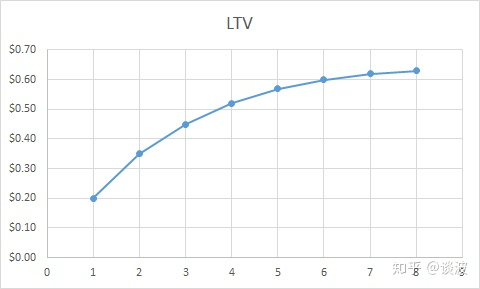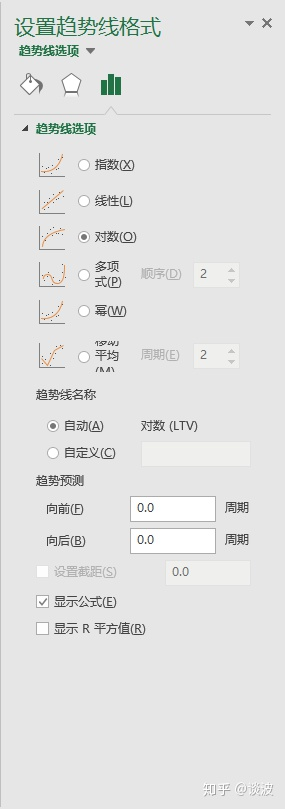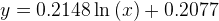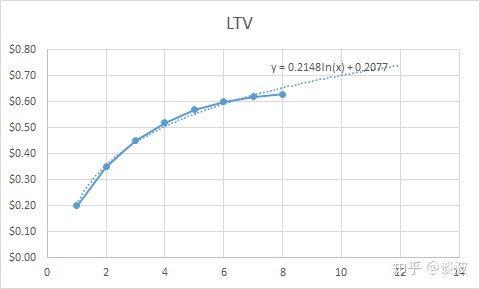Excel的趋势线工具提供了几个常用的函数，包括指数函数、对数函数等，可以满足一般需求。但是如果数据曲线相对复杂的话，就需要用到下面的工具了。

### Excel Solver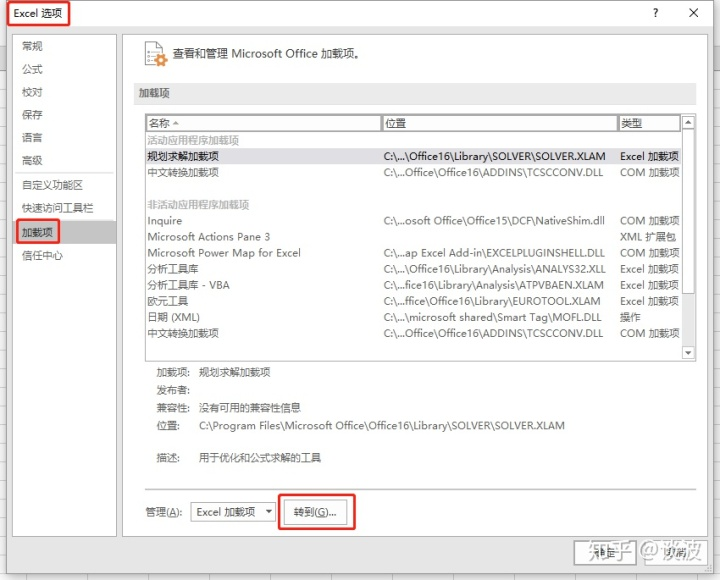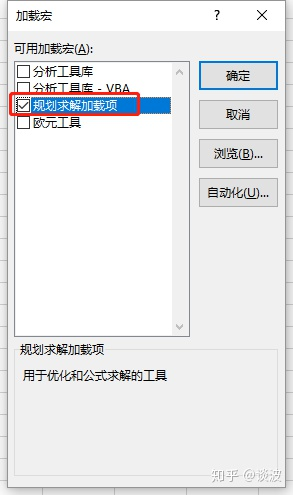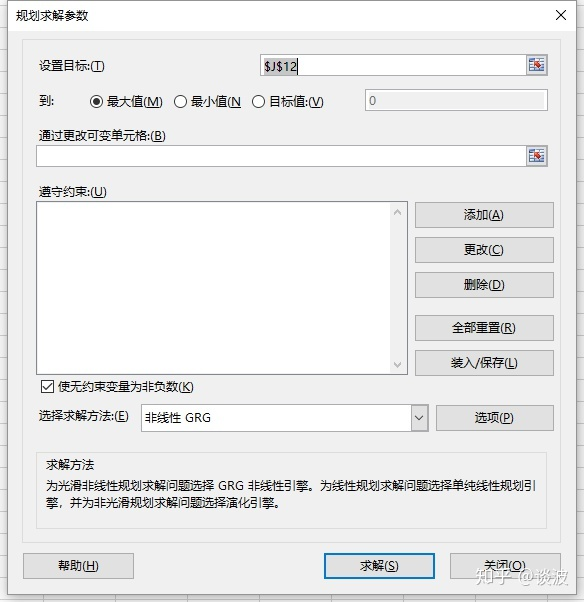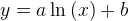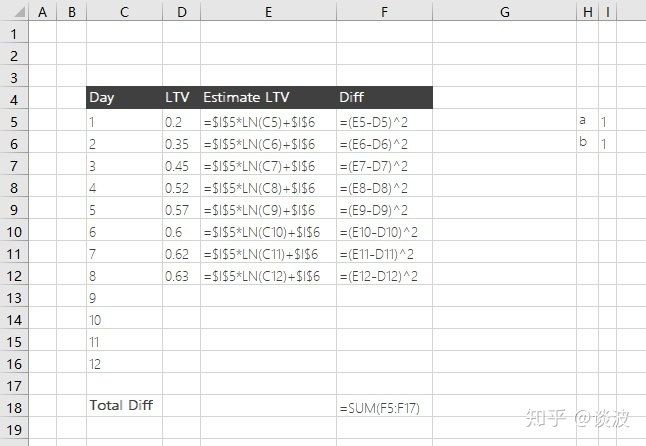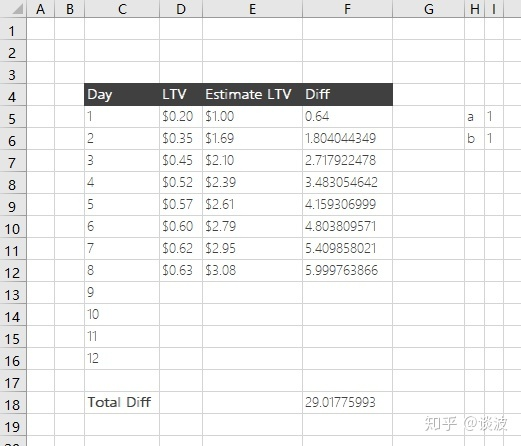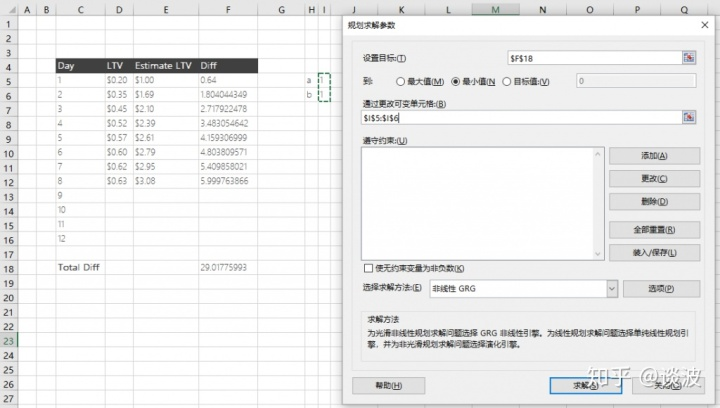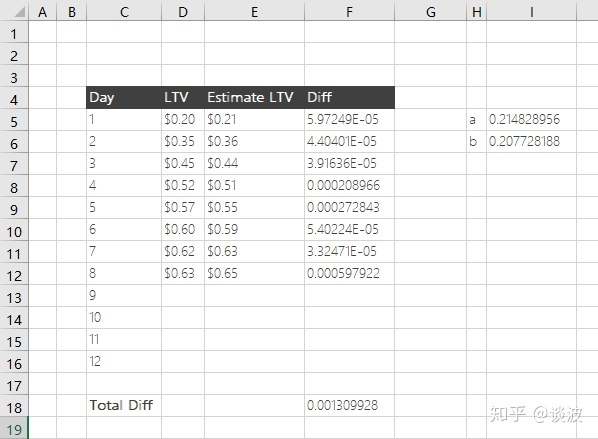### 在线工具

http://www.qinms.com/webapp/curvefit/cf.aspx

### Python matplotlib库

Python的matplotlib库有一个自定义公式来拟合曲线的功能。下面代码演示了通过它来拟合上述例子的过程

# -*- coding: UTF-8 -*-
import numpy as np
import matplotlib.pyplot as plt
from scipy import stats
import scipy.optimize as optimization

data_day = [1,2,3,4,5,6,7,8] #x坐标值|x coord
data_ltv = [0.2,0.35,0.45,0.52,0.57,0.6,0.62,0.63] #y坐标值|y coord

xdata = np.array(data_day)
ydata = np.array(data_ltv)

#定义使用的公式|customize equation
def lnFunction(x, A, B):
return A*np.log(x)+B

guess = [1, 1]  #定义初始A、B|initialize a and b
try:
params, params_covariance = optimization.curve_fit(lnFunction, xdata, ydata, guess) #拟合，A、B结果存入params|curve fitting and store a, b values to params
print params
result = '' #输出结果|to store result
for i in range(1, 15):
result += str(round(lnFunction(i, params, params), 2))    #将i带入公式中的x，使用拟合出的A、B值计算y值，并保留两位小数|calculate result for each i as x using the a, b values, and round the result to 2 points
if i != 14:
result += ','   #每个结果用逗号隔开，并省略最后一个逗号|separate each result with comma, and omit the last comma
print result
except:
print ''

[0.21482987 0.20772681]
0.21,0.36,0.44,0.51,0.55,0.59,0.63,0.65,0.68,0.7,0.72,0.74,0.76,0.77

## 曲线拟合公式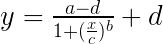### 四参数方程 – Solver比较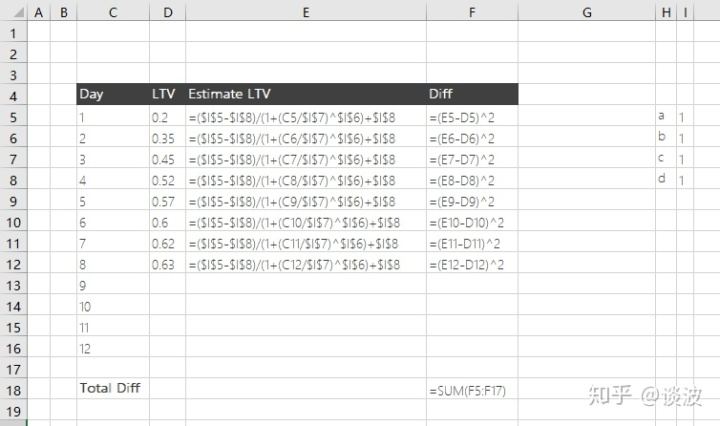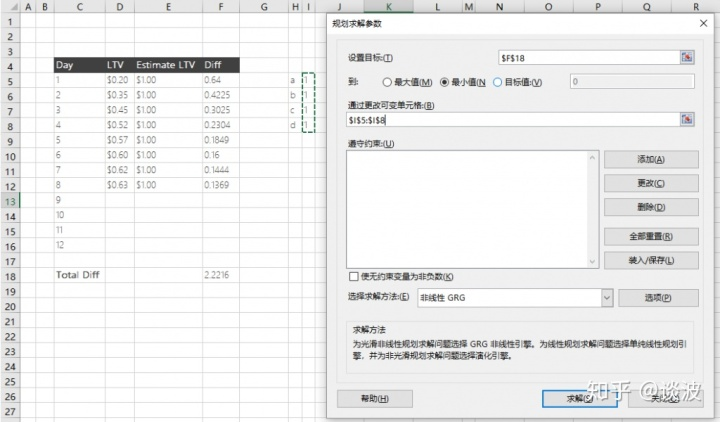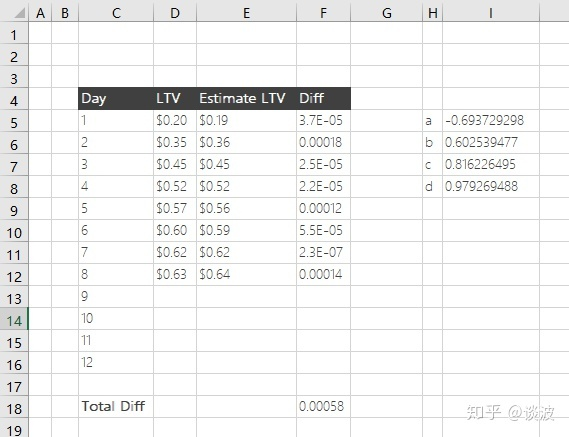### 四参数方程 – matplotlib比较

# -*- coding: UTF-8 -*-
import numpy as np
import matplotlib.pyplot as plt
from scipy import stats
import scipy.optimize as optimization

data_day = [1,2,3,4,5,6,7,8] #x坐标值|x coord
data_ltv = [0.2,0.35,0.45,0.52,0.57,0.6,0.62,0.63] #y坐标值|y coord

xdata = np.array(data_day)
ydata = np.array(data_ltv)

#定义使用的公式|customize equation
def fourPL(x, A, B, C, D):
return ((A-D)/(1.0+((x/C)**(B))) + D)

guess = [1, 1, 1, 1]  #定义初始A、B值|initialize a and b
param_bounds = ([-np.inf,-np.inf,0.001,-np.inf],[np.inf,np.inf,np.inf,np.inf])
try:
params, params_covariance = optimization.curve_fit(fourPL, xdata, ydata, guess, bounds = param_bounds) #拟合，A、B、C、D结果存入params|curve fitting and store a, b, c, d values to params
print params
result = '' #输出结果|to store result
for i in range(1, 15):
result += str(round(fourPL(i, params, params, params, params), 2))    #将i带入公式中的x，使用拟合出的A、B、C、D值计算y值，并保留两位小数|calculate result for each i as x using the a, b, c, d values, and round the result to 2 points
if i != 14:
result += ','   #每个结果用逗号隔开，并省略最后一个逗号|separate each result with comma, and omit the last comma
print result
except:
print ''

[0.0923509  1.73652154 2.41689489 0.70144044]
0.2,0.35,0.45,0.52,0.57,0.6,0.62,0.63,0.65,0.65,0.66,0.67,0.67,0.67

01-1303-19
08-2417万+
03-127296
07-21
10-295414
03-21447
02-28233
11-0230
©️2020 CSDN 皮肤主题: 数字20 设计师:CSDN官方博客